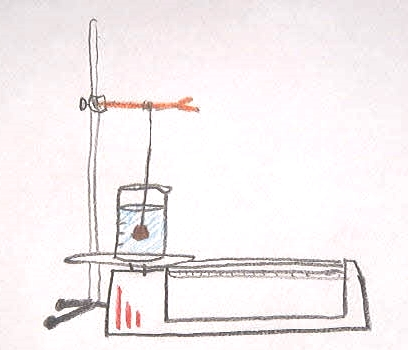## Doing Science: Buoyancy

### Sample Report#### Doing Science Questions

1. In this demonstration we weighed an object to find its mass. We used a rubber stopper. Then we weighed a beaker full of water. Next we tied the rubber stopper to a piece of string and let the stopper hang in the water on the balance. When we weighed it again the balance showed that some mass was added but the mass that was added was less than the mass of the stopper alone. If you look at the drawing you can see how we made this measurement.
2. The rubber stopper weighed 6.6 g. When suspended in the water it weighed 4.8 g after we subtracted the mass of the beaker and water. We thought that suspending something in water made it lose 1.8 g, or the difference between the two masses. To test this we tried using a brass weight with a mass of 100 g. Its suspended mass was only 11.7 g, which is a lot more than 1.8 g less than its actual mass. The difference is 88.3 g.
Next we thought the volume of the object might be what mattered. To test this idea we put the rubber stopper into a graduated cylinder with some water in it. By looking at the difference in the volume of the water before and after adding the stopper we found that it had a volume of 4.8 mL. We measured the volume of the brass weight the same way and found its volume to be 11.9 mL. Since these numbers were so similar to the suspended weights in grams we thought we might be on to something. Perhaps the volume in milliliters of an object gives its suspended mass in grams. To test the idea we measured the volume of an eraser (12.8 mL) and its suspended mass (12.8 g). This supported our idea.
3. Our final hypothesis is this:
If you suspend an object in water then the mass measured on a balance supporting the container and the water will increase by an amount in grams equal to the volume of the object in mL. The suspended mass of a 12.8 mL eraser was 12.8 grams. The suspended mass of the 100 g weight was 11.7 grams and its volume of 11.9 mL. While this is not exactly the same number it is so close that we think the difference is due to measurement error and not because there is some other explanation.
4. The drawing is at left.

#### Thought Questions

1. Imagine a string supports a cylinder suspended in water. The cylinder has a mass of 100 g. The balance reads 10 g after the mass of the beaker and water is subtracted. How much weight does the string support? Write one sentence justifying your answer.

The string supports 90 g. The mass of the cylinder doesn’t change. The string supports most of the weight and the water supports 10 grams of that weight.

2. A rubber stopper has a mass of 10 g. When placed under water in a graduated cylinder it is found that its volume is 8.0 mL. Next, the stopper is suspended from a string into a beaker full of water set up on a balance. What mass will the balance read after the mass of the beaker and the water is subtracted out?

The balance will give a suspended mass of the rubber stopper of 8.0 g. The volume of the stopper is 8.0 mL and the suspended mass equals the mass of the water that would take up the volume of the object. Since water has a density of 1 gram for every milliliter the suspended weight will be 8.0 g.

3. What if it wasn’t just plain water? Take an object that has a suspended mass of 10 g in water. What would its suspended mass be in salt water, with a density of 1.1 g/mL?

If the suspended mass is 10 g in water then the volume of the object must be 10 mL. Since the salt water is more dense (each milliliter is worth 1.1 grams instead of 1 gram) it will support more of the object’s weight. It will support 10 mL × 1.1 g/1 mL = 11 g.
This sample report replies to the questions in Doing Science and the additional questions added on for this experiment.

Last updated: Mar 16, 2017       Home